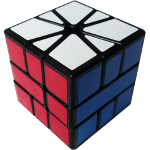### Square 1This puzzle has banding on the E layer so there are only two pieces on that layer making the only R or L moves possible 180° turns. The top and bottom layer have edges (30° wedges) and corners (60° wedges). The algorithm notation differs on this cube because of these changes so instead of R moves you have "/" a slash and U and D turns are number of 30° clockwise rotations so the algorithms look like: (1,0)/(-1,-1)/(0,1) which is U30° R2 U' D' R2 D

Return the puzzle to a cube shape.
Orient all corners.
Edge Swap:  (0,-1) / (-3,0) / (4,1) / (-4,-1) / (3,0) / (0,1)
Permute Corners: / (3,-3) / (3,0) / (-3,0) / (0,3) / (-3,0) /
Permute Edges: (1,0) / (0,3) / (-1,-1) / (1,-2) / (-1,0)
Permute Edge Parity: / (-3,0) / (0,3) / (0,-3) / (0,3) / (2,0) / (0,2) / (-2,0) / (4,0) / (0,-2) / (0,2) / (-1,4) / (0,-3) /
Swap Layers: / (6,-6) / (-1,1)
Orient the Center: / (6,0) / (6,0) / (6, 0)